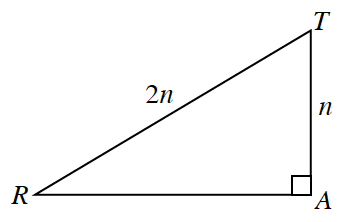### Home > PC3 > Chapter 2 > Lesson 2.2.5 > Problem2-119

2-119.​​Use $▵RAT$ at right complete the following problems.

1. Determine the exact length of $\overline{RA}$ in terms of $n$. What type of triangle is this?

Use the Pythagorean Theorem.

2. Using the triangle, write the ratios for:

1. $\sin(R)$

2. $\cos(R)$

3. $\tan(R)$

$\sin\left(R\right)=\frac{n}{2n}$

3. Use your results from part (b) to simplify $\frac { \operatorname { sin } ( R ) } { \operatorname { cos } ( R ) }$. What do you notice?

It is the same as $\tan(R)=\frac{1}{\sqrt{3}}=\frac{\sqrt{3}}{3}$.